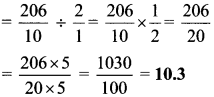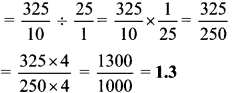# Maharashtra Board Practice Set 17 Class 6 Maths Solutions Chapter 5 Decimal Fractions

Balbharti Maharashtra State Board Class 6 Maths Solutions covers the Std 6 Maths Chapter 5 Decimal Fractions Class 6 Practice Set 17 Answers Solutions.

## 6th Standard Maths Practice Set 17 Answers Chapter 5 Decimal Fractions

Question 1.
Carry out the following divisions.
i. 4.8÷2
ii. 17.5÷5
iii. 20.6÷2
iv. 32.5÷25
Solution:
i. 4.8÷2ii. 17.5÷5iii. 20.6÷2iv. 32.5÷25Question 2.
A road is 4 km 800 m long. If trees are planted on both its sides at intervals of 9.6 m, how many trees were planted?
Solution:
Length of road = 4 km 800 m
= 4 × 1000 m + 800 m
= 4000 m + 800 m
= 4800 m
Number of trees on one side = 4800 ÷ 9.6= 500
∴ Number of trees on both sides = 2 x number of trees on one side
= 2 x 500 = 1000
If the trees are planted at the beginning of the road, then
Total number of trees = 1000 + 2 = 1002
∴ Total number of trees planted is 1000 or 1002.

Question 3.
Pradnya exercises regularly by walking along a circular path on a field. If she walks a distance of 3.825 km in 9 rounds of the path, how much does she walk in one round?
Solution:
Total distance walked in 9 rounds = 3.825 km
∴Distance walked in 1 round = 3.825 4 ÷ 9= 0.425 km
∴ Total distance walked in 1 round is 0.425 km.

Question 4.
A pharmaceutical manufacturer bought 0.25 quintal of hirada, a medicinal plant, for Rs 9500. What is the cost per quintal of hirada? (1 quintal = 100 kg)
Solution:
Cost of 0.25 quintal of hirada = Rs 9500
∴ Cost of 1 quintal of hirada = 9500 ÷ 0.25= Rs 38,000
∴ Cost per quintal of hirada is Rs 38,000.

#### Maharashtra Board Class 6 Maths Chapter 4 Operations on Fractions Practice Set 17 Intext Questions and Activities

Question 1.
Maths is fun! (Textbook pg. no. 34)

1. Consider any three-digit number (say 527).
2. Multiply the number by 7. Then multiply the product obtained by 13, and this product by 11.
3. The found product is 5,27,527.

Take two or three other numbers. Do the same multiplication and find out how it is done.
Solution:
7 × 13 × 11 = 1001
∴ 527 × 1001 = 527 × (1000+ 1)
= (527 × 1000) + (527 × 1)
= 527000 + 527 = 527527
Thus, when any three-digit number is multiplied with 1001, the product obtained is a six-digit number in which the original three-digit number is written back to back twice.
(Students may consider any other three-digit numbers and verify the property.)

Scroll to Top